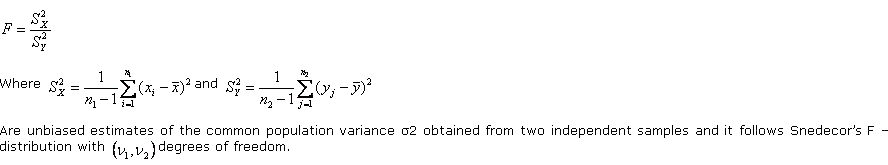# Statistics Assignment Help With F Test For Equality Of Population Variances

## 12.5.2. F test for equality of population variances

Suppose we want to test(i) whether two independent samples xi, (i = 1, 2, …..,n1) and yj, (j = 1, 2, …n2­) have been drawn from the normal populations with the same variance σ2, or (ii) whether the two independent estimates of the population variance are homogeneous or not.

Under the null hypothesis (H0) that (i) σX2 = σY2 = σ2 , that is the population variances are equal or (ii) two independent estimates of the population variance are homogeneous, the statistic F is given by

###### Email Based Homework Help in F Test For Equality Of Population Variances

To submit F Test For Equality Of Population Variances assignment click here.

### Following are some of the topics in F Test For Equality Of Population Variances in which we provide help:

Online Statistics Help | Statistics Math Help | Statistics probability help | Statistics help | College statistics help | Business statistics help | Elementary statistics help | Probability and statistics help | Statistics tutor | Statistic Homework help | Excel help | Mathematics help | Matlab help | MegaStat help | Minitab help | PHStat2 help | POM/QM help | R code and S-Plus help | SAS help | SPSS Help | Stata help | TDISK help | Tree Plan help | Online Tutoring